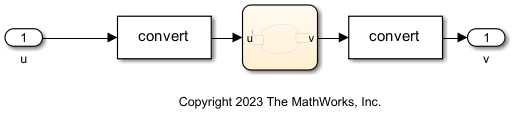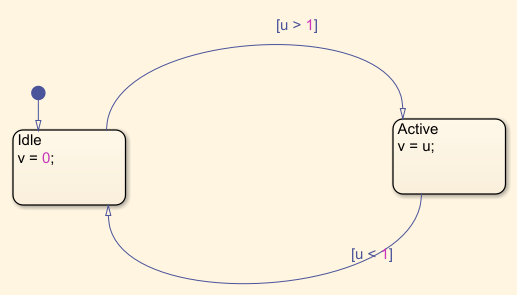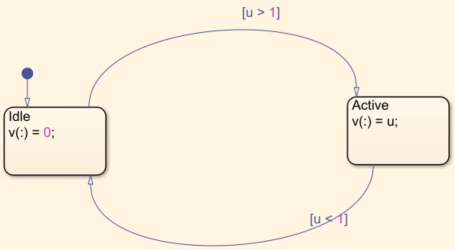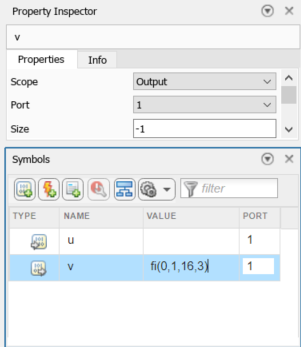## Best Practices for Working with Stateflow Charts in Automated Fixed-Point Conversion Workflows

Follow these best practices when using Stateflow® charts that use MATLAB® as the action language with automated fixed-point conversion workflows, including the Fixed-Point Tool.

### Issue: Stateflow Errors After Fixed-Point Conversion

After converting a model containing a Stateflow chart to use fixed-point data types, it is common to experience update diagram errors. These errors are often related to data type mismatches that were not caught during the conversion process. This simple example demonstrates symptoms of this issue.

#### Open and Run Simple Stateflow Model

The `simpleStateflowModel` contains a Stateflow chart surrounded by Data Type Conversion blocks.

```mdl = 'simpleStateflowModel'; open_system(mdl)```Inspect the Stateflow chart.

`open_system([mdl '/Chart'])`Simulate the model to ensure it runs without error.

`sim(mdl)`
```ans = Simulink.SimulationOutput: tout: [51x1 double] yout: [1x1 Simulink.SimulationData.Dataset] SimulationMetadata: [1x1 Simulink.SimulationMetadata] ErrorMessage: [0x0 char] ```

#### Apply Fixed-Point Data Types and Observe Update Diagram Errors

The Stateflow chart contains data objects `u` and `v`.

```chartData = find(sfroot,'-isa','Stateflow.Data',Path=[mdl '/Chart']); chartData.Name```
```ans = 'u' ans = 'v'```

If you apply different fixed-point data types to the variables `u` and `v` in the Stateflow chart, simulating the model results in update diagram errors. For example, set the data type of `u` to `fixdt(1,16,2)` and the data type of `v` to `fixdt(1,16,3)` and simulate the model.

```chartData(1).DataType = 'fixdt(1,16,2)'; chartData(2).DataType = 'fixdt(1,16,3)'; sim(mdl);```
```Compilation of model 'mStateflow' failed while trying to resolve underspecified signal dimensions. Suggested Actions: • Enable 'warning' or 'error' diagnostics for the list of underspecified signal dimensions. - Open Caused by: Error while generating code for chart Chart. Error in port widths or dimensions. 'Output Port 1' of 'mStateflow/Chart/u' is a one dimensional vector with 1 elements.```The source of the error is related to `u` and `v` having different data types. Stateflow additionally requires that the constant `0` assigned to `v` in the state `Idle` also agree with the data type of `v`.

### Best Practice: Separate Data Types from Algorithms in Stateflow Charts

In the simple example above, you could manually resolve the data type mismatch issues. However, this can be a difficult problem to resolve for large models with many mismatched data types. Rather than resolve these data type mismatch issues step by step, the best approach is to write your algorithms in such a way that they are agnostic to data type choices. Data type agnostic code has few, if any, constraints between data types. This allows you to easily experiment with different data type choices to understand the numerical effects of each data type choice. By removing the constraint that different variables and expressions must have the same data type, you open up a larger numerical space to explore. This approach allows your algorithms to operate with both floating-point and fixed-point data types, and is compatible with the automated fixed-point conversion tools in Fixed-Point Designer™, such as the Fixed-Point Tool.

Typically in MATLAB, reassigning a variable using the equal operator overwrites the contents of the variable. As a result, both the data and the data type of the variable are changed. For example, define the variable `a` to have a value of `1` and the default double-precision data type. When you use the equal operator to reassign the variable, the value and data type both change.

```a = 1; a = single(2)```
```a = single 2```

The `(:)=` syntax, equivalent to using the `subsasgn` function, is known as subscripted assignment. When you use this syntax, MATLAB overwrites the value of the left-hand side argument, but retains the existing data type and array size. For example, you can use `A(:)=B` to cast a value with one numeric type into another numeric type. This subscripted assignment statement assigns the value of `B` into `A` while keeping the numeric type of `A`. Subscripted assignment can be used for both fixed-point and integer data types, and for both scalar and array data.

Alternatively, when a variable is first defined, you can use the functions `cast`, `eye`, `ones`, or `zeros` to cast it to your desired data type. For more details, see Separate Data Type Definitions from Algorithm.

For example, define the variable `a` to be an array of values with a single-precision data type. Then use subscripted assignment to change the value of entries in the array `a` without changing the data type.

```a = single([1,2,3,4]); a(1) = 0 a(:) = 100```
```a = 1×4 single row vector 0 2 3 4 a = 1×4 single row vector 100 100 100 100```

In the `simpleStateflowModel` example, update the Stateflow chart as shown to use subscripted assignment.### Best Practice: Initialize Data in Stateflow Charts

To determine both the value and size of a variable, Stateflow requires an explicit specification for the initial value of variables. For detailed information on how to specify the initial value for data in Stateflow, see Set Data Properties (Stateflow).

To initialize data In the `simpleStateflowModel` example, on the Symbols pane, specify an initial value of `fi(0,1,16,3)` for the variable `v`. Alternatively, you could specify an initial value on the Property Inspector pane.With these changes, you can now compile and run the `simpleStateflowModel` example without errors.

### Stateflow Preparation Checks in the Fixed-Point Tool

Since R2023b

The Fixed-Point Tool includes preparation checks to ensure that assignments inside of Stateflow charts are casted and that all data are given an initial value. You should specify the data in terms of your initial data type; the Fixed-Point Tool will then propose a new data type during the conversion process. For more information, see Preparation Checks. When you apply proposed data types to the model, the initial value is updated by the Fixed-Point Tool to match the proposed data type.